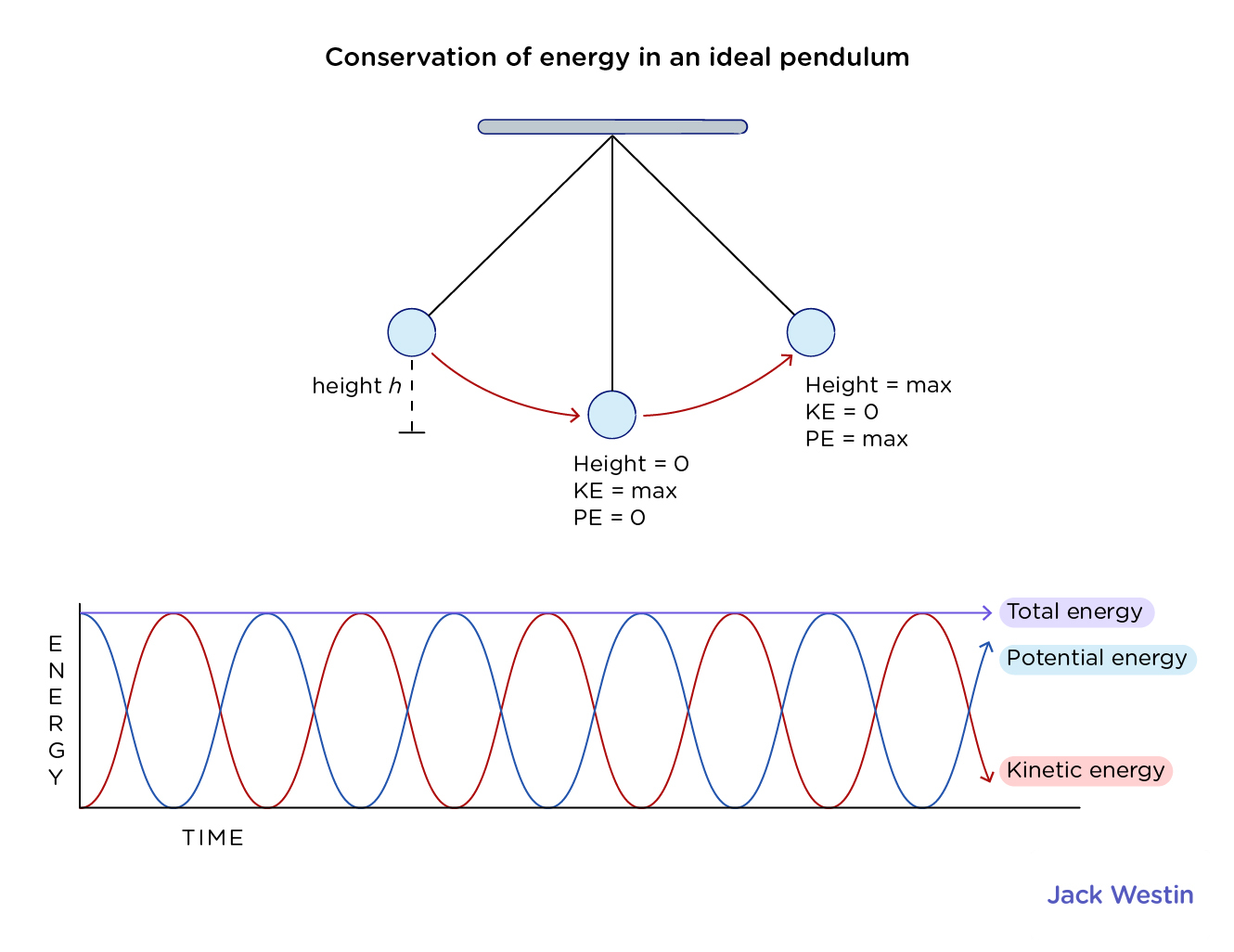Cyber Sale - Only 3 Days Remaining: ALL MCAT Products & Admissions Services

MCAT Content / Energy Of Point Object Systems / Conservation Of Energy

### Conservation of energy

Topic: Energy Of Point Object Systems

Conservation of mechanical energy states that the mechanical energy of an isolated system remains constant without friction.

According to Work – Kinetic Energy theorem:

Wnet = ΔKE where Wnet is the net work in a system and delta KE is the change in kinetic energy.

If only conservative forces act, then Wnet=Wc, where Wc is the total work done by all conservative forces. Thus, Wc = ΔKE. Now, if the conservative force, such as the gravitational force or a spring force, does work, the system loses potential energy (PE). That is, Wc = −PE. Therefore,

−ΔPE = ΔKE

This equation means that the total kinetic and potential energy is constant for any process involving only conservative forces. That is,

KE + PE = constant

In any real situation, frictional forces and other non-conservative forces are always present, but in many cases, their effects on the system are so small that the principle of conservation of mechanical energy can be used as a fair approximation. Though energy cannot be created nor destroyed in an isolated system, it can be internally converted to any other form of energy.Practice Questions

Understanding cardiac pressure-volume curves

Your heart does work: A relationship of pressure and volume

Rollercoasters are energy at work!

MCAT Official Prep (AAMC)

Physics Online Flashcards Question 14

Physics Question Pack Passage 6 Question 39

Physics Question Pack Passage 7 Question 46

Physics Question Pack Passage 15 Question 87

Section Bank C/P Section Passage 2 Question 8

Practice Exam 2 C/P Section Question 57

Key Points

• The conservation of mechanical energy can be written as “KE + PE = const”.

• Though energy cannot be created nor destroyed in an isolated system, it can be internally converted to any other form of energy.

Key Terms

Conservation: a particular measurable property of an isolated physical system does not change as the system evolves.

Frictional force: frictional force is the force resisting the relative motion of solid surfaces, fluid layers, and material elements sliding against each other.

Isolated system: a system that does not interact with its surroundings, that is, its total energy and mass stay constant

Kinetic energy: kinetic energy of an object is the energy that it possesses due to its motion

Conservative force: a force with the property that the total work is done in moving a particle between two points is independent of the taken path

Billing Information